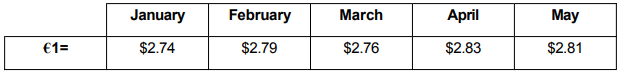# IMAT 2012 Q18 [Currency Exchange]

In preparation for my holiday in Bolandia last June, I changed €300 into Bolandian dollars every pay day from January to May (I am paid monthly).

The exchange rates were as follows:How many more Bolandian dollars would I have received altogether if, instead, I had changed the whole €1500 in May?

A. 36
B. 48
C. 66
D. 180
E. 105

Simple steps to solve word problems:

• Underline key information
• Determine what they are trying to ask, and what you will need to solve it
Eliminate any non-essential information
• Draw a picture, graph, or equation
In moments of high stress like exam taking, always work with the paper they give you to avoid careless mistakes.
• Solve.

The first thing we want to do is to notice that there is a reasonable gap between the answers, meaning we can round and still keep a certain degree of accuracy if we need to. This is another question that requires a decent level of multiplication.

A trick: Google how to solve long multiplication (ex. Three digits times three digits). Using 2.74 as an example, you can multiply it by 100 to get 274 and then it is easier to do 274 times 300 and then finally divide your answer by 100 (take away two decimal place). Find a trick that works for you.

Approach: First find out the total amount you will receive if you multiply by the exchange rate and then sum it. Next find out how much you would get if you just used the May exchange rate. Finally find the difference.

1. Find the total amount if you multiply the exchange rates from every month by €300

Jan: (2.74)(€300)=822

Feb: (2.79)(€300)=837

March: (2.76)(€300)=828

April: (2.83)(€300)=849

May: (2.81)(€300)=843

Total: 822+837+828+849+843=4179

1. Find the amount if you transferred all in May.

(2.81)(€1500)=4215

1. Find the difference

4215-4179=36

As you can see, this was very long and time consuming, so what are some faster solutions? Take the average of the 5 different exchange rates and then multiply it by 1500.

$$\frac{\text{2.74+2.79+2.76+2.83+2.81}{5}$$

$$\frac{\text{13.93}{5}$$

If you analyse this, you can figure out that it is roughly = 2.79. You should be able to do this on paper, find a trick that works for you.

(2.79)(€1500)=4179

And then continue using the same method above.